# Heisenberg's uncertainty principle facts for kids

Kids Encyclopedia Facts

Heisenberg's uncertainty principle is one of the most important results of twentieth century physics. It relates to measurements of sub-atomic particles. Certain pairs of measurements such as (a) where a particle is and (b) where it is going (its position and momentum) cannot be precisely pinned down.p96

Albert Einstein thought that such a quantum theory could give us only a partial description of nature.p99 He thought that Heisenberg's discovery showed that human knowledge is limited, but he also thought that nature is absolute. That is, he thought that there is no "uncertainty" in nature, and that the uncertainty exists only in our knowledge about it. However, many other scientists disagree with Einstein.

Heisenberg's idea is that if something like an electron were shot into a big box with a certain speed and in a certain direction, then it would be possible to calculate a fairly accurate idea of what its path would be like in the future. However, if the box were made smaller then we would have a more certain idea of where it was located, and because of that we would have to have a less certain idea of how it was traveling. American physicist Brian Greene gave the analogy of a moth which flies placidly around in a large closet but who flies frantically back and forth and up and down when placed in a glass jar.p114 Another interesting uncertainty phenomenon, which makes possible many electronic devices, is called quantum tunneling. In our everyday life, people cannot move through walls. However, electrons can move through solid walls.p115 In the animation to the right, you can see a faint white puff on the right side of the wall after a big puff hits the wall from the left. That dim spot of light represents the photon or other atomic particle which tunnels through the wall.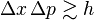$\Delta x \, \Delta p\gtrsim h\qquad\qquad\qquad$
The minimum range of error in position (x) times the minimum range of error in momentum (p) is, at a minimum, about equal to the Planck constant.

## Confusion with observer effect

Historically, the uncertainty principle has been confused with a somewhat similar effect in physics, called the observer effect. This says that measurements of some systems cannot be made without affecting the systems. Heisenberg offered such an observer effect at the quantum level as a physical "explanation" of quantum uncertainty.

However, it is now clear that the uncertainty principle is a property of all wave-like systems. It arises in quantum mechanics simply due to the matter wave nature of all quantum objects. Thus, the uncertainty principle actually states a fundamental property of quantum systems, and is not a statement about the observational success of current technology. "Measurement" does not mean just a process in which a physicist-observer takes part, but rather any interaction between classical and quantum objects regardless of any observer.

## The idea of indeterminacy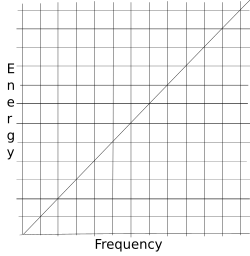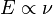$E \propto \nu$,
i.e., Energy is proportional to frequency

The uncertainty principle came from Werner Heisenberg's matrix mechanics. Max Planck already knew that the energy of a unit of light is proportional to the frequency of that unit of light ($E \propto \nu$), and that its amount of energy can be expressed in familiar terms such as the joule by using a proportionality constant. The constant he gave the world is now called the Planck constant and is represented by the letter h. When matrices are used to express quantum mechanics, frequently two matrices have to be multiplied to get a third matrix that gives the answer the physicist is trying to find. But multiplying a matrix such as P (for momentum) by a matrix such as X (for position) gives a different answer matrix from the one you get when you multiply X by P. The result of multiplying P by X and X by P and then comparing them always involves the Planck constant as a factor. The number used to write the Planck constant will always depend on the system of measurement in use. (With a certain system of measurement, its numerical value is one.) The slope of the line in the diagram to the right that shows the ratio of frequency to energy will also depend on the system of measurement chosen.

The following diagrams show what happens when we try to measure both location and momentum.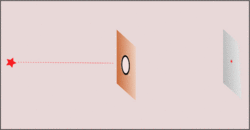Narrowing the gap increases the certainty of where the photon is in the middle, but then its direction from there to the detection screen on the right becomes correspondingly more uncertain.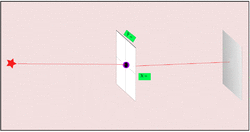Suspending the center gap by springs lets momentum be measured, but unpredictably moves the gap so information on the photon's location in the middle gets lost.

The practical result of this mathematical discovery is that when a physicist makes position more clear then momentum becomes less clear, and that when the physicist makes momentum more clear then position becomes less clear. Heisenberg said that things are "indeterminate," and other people liked to say that they were "uncertain." But the mathematics shows that it is the things in the world that are indeterminate or "fuzzy," and not that it is just that humans are uncertain about what is going on.

## Putting indeterminacy into mathematical form

Here we will show the first equation that gave the basic idea later shown in Heisenberg's uncertainty principle.

Heisenberg's groundbreaking paper of 1925 does not use and does not even mention matrices. Heisenberg's great success was the "scheme which was capable in principle of determining uniquely the relevant physical qualities (transition frequencies and amplitudes)" of hydrogen radiation.

After Heisenberg wrote his breakthrough paper, he gave it to one of his teachers to fix up and went on a vacation. Max Born was puzzled by the equations and the non-commuting equations that even Heisenberg thought were a problem. After several days Born realized that these equations were directions for writing out matrices. Matrices were new and strange, even for mathematicians of that time, but how to do math with them was already clearly known. He and a few others worked everything out in matrix form before Heisenberg came back from his time off, and within a few months the new quantum mechanics in matrix form gave them the basis for another paper.

Max Born saw that when the matrices that represent pq and qp were calculated they would not be equal. Heisenberg had already seen the same thing in terms of his original way of writing things out, and Heisenberg may have guessed what was almost immediately obvious to Born—that the difference between the answer matrices for pq and for qp would always involve two factors that came out of Heisenberg's original math: Planck's constant h and i, which is the square root of negative one. So the very idea of what Heisenberg preferred to call the "indeterminacy principle" (usually known as the uncertainty principle) was hiding in Heisenberg's original equations.

Heisenberg had been looking at changes that happen in an atom when an electron changes its energy level and so gets closer to the center of its atom or gets farther from its center, and, especially, situations in which an electron falls to a lower energy state in two steps. Max Born explained how he took Heisenberg's strange "recipe" for finding the product, C, of some change in an atom from energy level n to energy level n-b, which involved taking the sum of multiplying one change in something called A (which could be, for instance, the frequency of some photon) produced by a change of energy of an electron in the atom between energy state n and energy state n-b) by a succeeding change in something called B (which could be, for instance, the amplitude of a change) produced by another change in energy state from n-a to n-b):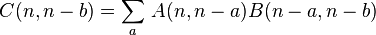$C(n,n-b) = \sum_{a}^{} \, A(n,n-a)B(n-a,n-b)$

and discovered something ground breaking:

By consideration of ...examples...[Heisenberg] found this rule.... This was in the summer of 1925. Heisenberg...took leave of absence...and handed over his paper to me for publication....

Heisenberg's rule of multiplication left me no peace, and after a week of intensive thought and trial, I suddenly remembered an algebraic theory....Such quadratic arrays are quite familiar to mathematicians and are called matrices, in association with a definite rule of multiplication. I applied this rule to Heisenberg's quantum condition and found that it agreed for the diagonal elements. It was easy to guess what the remaining elements must be, namely, null; and immediately there stood before me the strange formula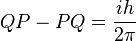${QP - PQ = \frac{ih}{2\pi}}$
[The symbol Q is the matrix for displacement, P is the matrix for momentum, i stands for the square root of negative one, and h is Planck's constant.]

Later on, Heisenberg put his discovery into another mathematical form: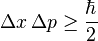$\Delta x\, \Delta p \ge \frac{\hbar}{2}$

(The special symbol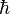${\hbar}$ is called "h-bar," and is equal to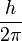${\frac{h}{2\pi}}$.)

The math is a way of describing things that happen in the real world. You might imagine that it would be easy to get both the exact position of something and its exact mass, path, and speed at the same time. However, in reality you have to do two things to get your answer. If you are measuring the position and momentum of a bullet that is stuck in a cliff of a great mountain somewhere, it is a simple matter. The mountain does not appear to be going anywhere, and neither is the bullet. So its position is known and its velocity is 0, so its momentum is also 0. But if the bullet is somewhere between a gun and a target, it will be difficult to get its position at any given time. The best we may be able to do is to take its picture using a camera with a very fast shutter. But a single squeeze of the shutter would give you only one thing, the bullet's position at time t. To get the momentum we might put a block of paraffin in the bullet's way and measure how the block of paraffin moved when it stopped the bullet. Or, if we knew the bullet's mass, we might take a sequence of two pictures, compute the velocity by knowing the difference between the two positions of the bullet and the time between its two appearances. However we do it, we need to measure mass and position and time between appearances. We end up making at least two measurements to get to x and p. In that case we have to choose which measurement to make first, and which to make second. It seems to make no difference which order our measurements are made in. Measuring the bullet's mass and then measuring its positions twice, or measuring the bullet's positions twice and then recovering the bullet and measuring its mass would not make any difference, would it? After all, we have not done anything to the bullet when we weigh it or when we make photographs of it.

On the very small scale when we are measuring something like an electron, however, each measurement does something to it. If we measure position first, then we change its momemtum in the process. If we measure the electron's momentum first, then we change its position in the process. Our hope would be to measure one of them and then measure the other before anything changed, but our measuring itself makes a change, and the best we can hope to do is to reduce to a minimum the energy we contribute to the electron by measuring it. That minimum amount of energy has the Planck constant as one of its factors.

## Uncertainty goes beyond matrix math

Heisenberg's uncertainty principle was found in the earliest equations of the "new" quantum physics, and the theory was given by using matrix math. However, the uncertainty principle is a fact about nature, and it shows up in other ways of talking about quantum physics such as the equations made by Erwin Schrödinger.

## Indeterminacy in nature, not uncertainty of humans

There have been two very different ways of looking at what Heisenberg discovered: Some people think that things that happen in nature are "determinate," that is, things happen by a definite rule and if we could know everything we need to know we could always say what will happen next. Other people think that things that happen in nature are guided only by probability, and we can know only how things will behave on the average—but we know that very precisely.

The physicist John Stewart Bell discovered a way to prove that the first way cannot be correct. His work is called Bell's theorem or Bell's Inequality.

## Popular culture

The expression "quantum leap" or "quantum jump" has been taken to mean some great and transformative change, and it is often used in hyperbolic expressions by politicians and mass media sales campaigns. In quantum mechanics it is used to describe the transition of an electron from one orbit around the nucleus of an atom to any other orbit, higher or lower.

Sometimes the word "quantum" is used in the names of commercial products and businesses. For instance, Briggs and Stratton manufactures many kinds of small gasoline motors for lawn mowers, rotary tillers, and other such small machines. One of their model names is "Quantum."

Because the uncertainty principle tells us that certain measurements at the atomic level cannot be made without disturbing other measurements, some individuals use this idea to describe instances in the human world where the activity of an observer changes the thing that is being watched. An anthropologist may go to some far away place to learn how people live there, but the fact that a strange person from the outside world is there watching them may change the ways that those people act.

Things that people do while observing thing that change what is being observed are cases of the Observer effect. Some things that people do cause changes on the very small level of atoms and are cases of uncertainty or indeterminacy as first described by Heisenberg. The uncertainty principle shows that there is always a limit to how small we can make certain pairs of measurements such as position and speed or trajectory and momentum. The observer effect says that sometimes what people do in observing things, e.g., learning about an ant colony by digging it up with garden tools, can have big effects that change what they were trying to learn about.

## Images for kidsHeisenberg's uncertainty principle Facts for Kids. Kiddle Encyclopedia.